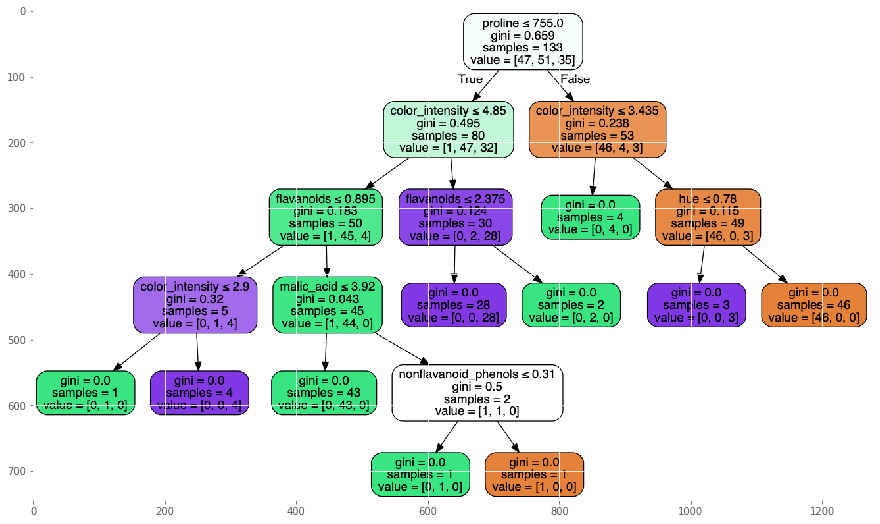How to visualise a tree model Multiclass Classification?
MACHINE LEARNING RECIPES DATA CLEANING PYTHON DATA MUNGING PANDAS CHEATSHEET     ALL TAGS

# How to visualise a tree model Multiclass Classification?

This recipe helps you visualise a tree model Multiclass Classification

0
In :
```## How to visualise a tree model - Multiclass Classification
def Snippet_180():
print()
print(format('How to visualise a tree model - Multiclass Classification','*^82'))

import warnings
warnings.filterwarnings("ignore")

from sklearn import datasets
from sklearn import metrics
from sklearn.model_selection import train_test_split
import matplotlib.pyplot as plt

plt.style.use('ggplot')

from sklearn import tree
from sklearn.externals.six import StringIO
import pydotplus

X = dataset.data; y = dataset.target
X_train, X_test, y_train, y_test = train_test_split(X, y, test_size=0.25)

# fit a tree.DecisionTreeClassifier() model to the data
model = tree.DecisionTreeClassifier()
model.fit(X_train, y_train)
print(); print(model)

# make predictions
expected_y  = y_test
predicted_y = model.predict(X_test)

# summarize the fit of the model
print(); print('tree.DecisionTreeClassifier(): ')
print(); print(metrics.classification_report(expected_y, predicted_y,
target_names=dataset.target_names))
print(); print(metrics.confusion_matrix(expected_y, predicted_y))

# Create a dot file
dotfile = open("tree.dot", 'w')
tree.export_graphviz(model, out_file = dotfile, feature_names = dataset.feature_names)
dotfile.close()

# Create pdf and png from the dot data
dot_data = StringIO()
tree.export_graphviz(model, out_file=dot_data,  filled=True, rounded=True,
special_characters=True, feature_names = dataset.feature_names)
graph = pydotplus.graph_from_dot_data(dot_data.getvalue())

graph.write_png("tree.png")
graph.write_pdf("tree.pdf")

import matplotlib.image as mpimg
plt.figure(figsize=(15,15))
plt.imshow(img)
plt.show()
Snippet_180()
```
```************How to visualise a tree model - Multiclass Classification*************

DecisionTreeClassifier(class_weight=None, criterion='gini', max_depth=None,
max_features=None, max_leaf_nodes=None,
min_impurity_decrease=0.0, min_impurity_split=None,
min_samples_leaf=1, min_samples_split=2,
min_weight_fraction_leaf=0.0, presort=False, random_state=None,
splitter='best')

tree.DecisionTreeClassifier():

precision    recall  f1-score   support

class_0       0.85      0.92      0.88        12
class_1       0.89      0.85      0.87        20
class_2       0.92      0.92      0.92        13

micro avg       0.89      0.89      0.89        45
macro avg       0.89      0.90      0.89        45
weighted avg       0.89      0.89      0.89        45

[[11  1  0]
[ 2 17  1]
[ 0  1 12]]
```#### Relevant Projects

##### Music Recommendation System Project using Python and R
Machine Learning Project - Work with KKBOX's Music Recommendation System dataset to build the best music recommendation engine.

##### Sequence Classification with LSTM RNN in Python with Keras
In this project, we are going to work on Sequence to Sequence Prediction using IMDB Movie Review Dataset​ using Keras in Python.

##### Solving Multiple Classification use cases Using H2O
In this project, we are going to talk about H2O and functionality in terms of building Machine Learning models.

##### Resume parsing with Machine learning - NLP with Python OCR and Spacy
In this machine learning resume parser example we use the popular Spacy NLP python library for OCR and text classification.

##### Choosing the right Time Series Forecasting Methods
There are different time series forecasting methods to forecast stock price, demand etc. In this machine learning project, you will learn to determine which forecasting method to be used when and how to apply with time series forecasting example.

##### Ensemble Machine Learning Project - All State Insurance Claims Severity Prediction
In this ensemble machine learning project, we will predict what kind of claims an insurance company will get. This is implemented in python using ensemble machine learning algorithms.

##### Forecast Inventory demand using historical sales data in R
In this machine learning project, you will develop a machine learning model to accurately forecast inventory demand based on historical sales data.

##### Machine Learning or Predictive Models in IoT - Energy Prediction Use Case
In this machine learning and IoT project, we are going to test out the experimental data using various predictive models and train the models and break the energy usage.

##### Mercari Price Suggestion Challenge Data Science Project
Data Science Project in Python- Build a machine learning algorithm that automatically suggests the right product prices.

##### Predict Macro Economic Trends using Kaggle Financial Dataset
In this machine learning project, you will uncover the predictive value in an uncertain world by using various artificial intelligence, machine learning, advanced regression and feature transformation techniques.